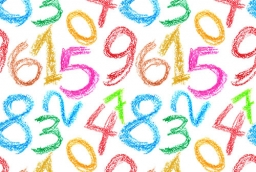# Determine 25341

In a two-digit number, the number of tens is three more than the number of ones. If we multiply the original number by a number written with the same digits but in the reverse order, we get the product 3 478. Determine the actual number.

x =  74

### Step-by-step explanation:Did you find an error or inaccuracy? Feel free to write us. Thank you!

Tips for related online calculators
Are you looking for help with calculating roots of a quadratic equation?
Do you have a linear equation or system of equations and looking for its solution? Or do you have a quadratic equation?